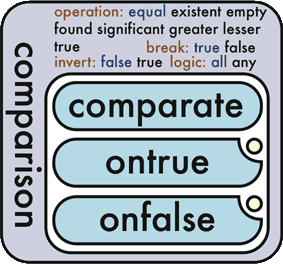### The Comparison Function

Comparison is a flow control function which allows a comparison to occur between one or more comparate values, and processes a defined true or [false] value.Comparison is commonly used for processing and validation of input data, or anywhere a decision must be made as to what the next action of the application should be.
• operation: Operation indicates the type of comparison which should be applied against the comparate values
• invert (false*/true): Invert gives the opposite result returned from the comparison
• logic (all*/any): logic (any) returns true if any comparate meets the criteria. The default (all) requires all comparates to meet the criteria.
break (true*/false): break (true) stops evaluating comparates as soon as the result is available. For instance, with an equal test over twenty comparates, as soon as there are two comparates that are not equal, the comparison operation no longer needs to continue to evaluate comparates in order to complete.)See also:
The following examples show the results of basic equal comparison
``````<comparison>
<comparate>green<@L(!docComparate)>
<comparate>green<@L(!docComparate)>
<ontrue>They're the same!</ontrue>
<onfalse>They're different!</onfalse>
</comparison>``````
`They're the same!`
Since thecomparates are both green, the true case is returned.

``````<comparison>
<comparate>green<@L(!docComparate)>
<comparate>blue<@L(!docComparate)>
<comparate>green<@L(!docComparate)>
<ontrue>They're the same!</ontrue>
<onfalse>They're different!</onfalse>
</comparison>``````
`They're different!`
Since thecomparates are not all the same (green, blue, green) the false case is returned.

``````<instruction>
<output type="store" value="myStore" />
<input>Contents!</input>
</instruction>
<comparison operation="existent">
<comparate type="store" value="myStore" />
<ontrue>myStore Exists!</ontrue>
<onfalse>myStore is only a figment of my imagination...</onfalse>
</comparison>``````
`myStore Exists!`

``````<instruction>
<output type="store" value="myStore" />
<input>Test test test</input>
</instruction>
<comparison operation="empty">
<comparate type="store" value="myStore" />
<ontrue>I'm empty</ontrue>
<onfalse>I'm full!</onfalse>
</comparison>``````
`I'm full!`

``````<c:comparison operation="significant">
<c:comparate value="cat" />
<c:ontrue>Yes I am</c:ontrue>
<c:onfalse>No I'm not</c:onfalse>
</c:comparison>``````
`Yes I am`

``````<c:comparison operation="found" note="assume that there IS a file called book.xml">
<c:comparate space="file" value=".ook\.xml" />
<c:ontrue value="true" />
<c:onfalse value="false" />
</c:comparison>``````
`true`
``````<c:comparison operation="lesser">
<c:comparate value="7" />
<c:comparate value="3" />
<c:comparate value="7" />
<c:ontrue value="seventh heaven" />
<c:onfalse value="seven sins" />
</c:comparison>``````
`seven sins`

``````<c:comparison operation="greater">
<c:comparate value="9" />
<c:comparate value="6" />
<c:ontrue value="Why yes, that is greater..." />
<c:onfalse value="Just plain appaling" />
</c:comparison>``````
`Why yes, that is greater...`
``````<comparison>
<comparate>100</comparate>
<ontrue>My o's are rounder than your 0's</ontrue>
<onfalse>I'm afraid not</onfalse>
</comparison>``````
`My o's are rounder than your 0's`